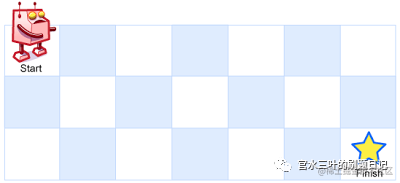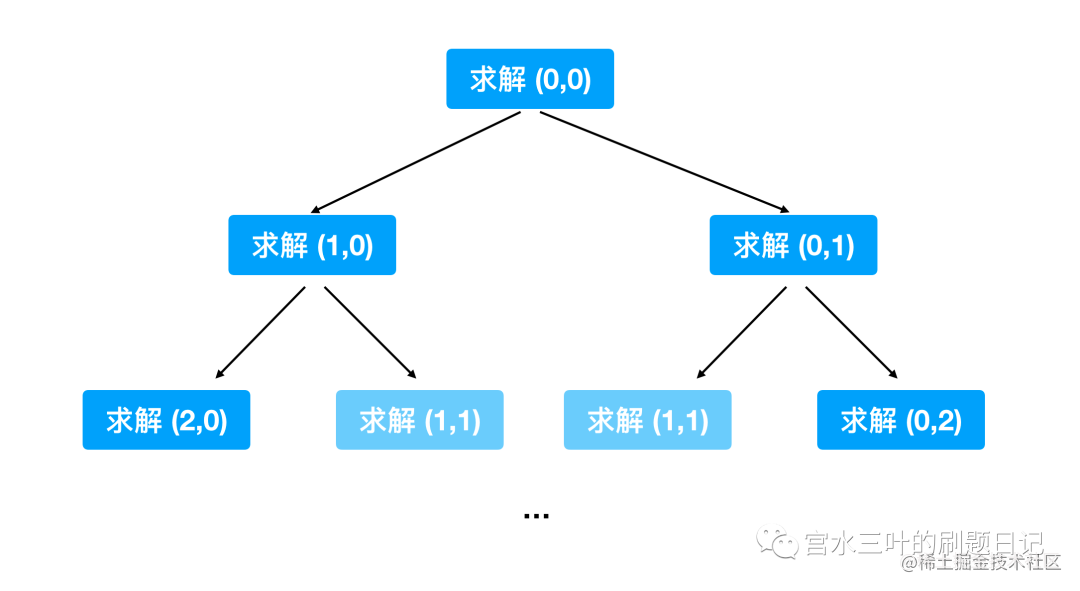# 一份给算法新人们的「动态规划」讲解

「本文已参与好文召集令活动，点击查看：后端、大前端双赛道投稿，2万元奖池等你挑战！

## 无后效性### 1：暴力递归

class Solution {
public int uniquePaths(int m, int n) {
return recursive(m, n, 0, 0);
}

private int recursive(int m, int n, int i, int j) {
if (i == m - 1 || j == n - 1) return 1;
return recursive(m, n, i + 1, j) + recursive(m, n, i, j + 1);
}
}

1. Base case: 由于题目明确了机器人只能往下或者往右两个方向走，所以可以定下来递归方法的 base case 是当已经处于矩阵的最后一行或者最后一列，即只一条路可以走。

2. 其余情况：机器人既可以往右走也可以往下走，所以对于某一个位置来说，到达右下角的路径数量等于它右边位置到达右下角的路径数量 + 它下方位置到达右下角的路径数量。即 recursive(m,n,i+1,j) + recursive(m,n,i,j+1)，这两个位置都可以通过递归函数进行求解。

### 2：记忆化搜索class Solution {
private int[][] cache;

public int uniquePaths(int m, int n) {
cache = new int[m][n];
for (int i = 0; i < m; i++) {
int[] ints = new int[n];
Arrays.fill(ints, -1);
cache[i] = ints;
}
return recursive(m, n, 0, 0);
}

private int recursive(int m, int n, int i, int j) {
if (i == m - 1 || j == n - 1) return 1;
if (cache[i][j] == -1) {
if (cache[i + 1][j] == -1) {
cache[i + 1][j] = recursive(m, n, i + 1, j);
}
if (cache[i][j + 1] == -1) {
cache[i][j + 1] = recursive(m, n, i, j + 1);
}
cache[i][j] = cache[i + 1][j] + cache[i][j + 1];
}
return cache[i][j];
}
}

### 2.1：次优解版本的「记忆化搜索」

class Solution {
private int[][] cache;

public int uniquePaths(int m, int n) {
cache = new int[m][n];
for (int i = 0; i < m; i++) {
int[] ints = new int[n];
Arrays.fill(ints, -1);
cache[i] = ints;
}
return recursive(m, n, 0, 0);
}

private int recursive(int m, int n, int i, int j) {
if (i == m - 1 || j == n - 1) return 1;
if (cache[i][j] == -1) {
cache[i][j] = recursive(m, n, i + 1, j) + recursive(m, n, i, j + 1);
}
return cache[i][j];
}
}

class Solution {

public static void main(String[] args) {
Solution solution = new Solution();
solution.uniquePaths(15, 15);
}

private int[][] cache;
private long count; // 统计 递归函数 的调用次数

public int uniquePaths(int m, int n) {
cache = new int[m][n];
for (int i = 0; i < m; i++) {
int[] ints = new int[n];
Arrays.fill(ints, -1);
cache[i] = ints;
}
// int result = recursive(m, n, 0, 0); // count = 80233199
// int result = cacheRecursive(m, n, 0, 0); // count = 393
int result = fullCacheRecursive(m, n, 0, 0); // count = 224
System.out.println(count);
return result;
}

// 完全缓存
private int fullCacheRecursive(int m, int n, int i, int j) {
count++;
if (i == m - 1 || j == n - 1) return 1;
if (cache[i][j] == -1) {
if (cache[i + 1][j] == -1) {
cache[i + 1][j] = fullCacheRecursive(m, n, i + 1, j);
}
if (cache[i][j + 1] == -1) {
cache[i][j + 1] = fullCacheRecursive(m, n, i, j + 1);
}
cache[i][j] = cache[i + 1][j] + cache[i][j + 1];
}
return cache[i][j];
}

// 只有外层缓存
private int cacheRecursive(int m, int n, int i, int j) {
count++;
if (i == m - 1 || j == n - 1) return 1;
if (cache[i][j] == -1) {
cache[i][j] = cacheRecursive(m, n, i + 1, j) + cacheRecursive(m, n, i, j + 1);
}
return cache[i][j];
}

// 不使用缓存
private int recursive(int m, int n, int i, int j) {
count++;
if (i == m - 1 || j == n - 1) return 1;
return recursive(m, n, i + 1, j) + recursive(m, n, i, j + 1);
}
}

### 3：从「自顶向下」到「自底向上」

$f(n) =\begin{cases} 1 & n = 1, 2 \\ f(n - 1) + f(n - 2) & n > 2 \end{cases}$

public class Solution {
private int[] cache;
public int fib(int n) {
cache = new int[n + 1];
return recursive(n);
}

private int recursive(int n) {
if (n <= 1) return n;
if (n == 2) return 1;
if (cache[n] == 0) {
if (cache[n - 1] == 0) {
cache[n - 1] = recursive(n - 1);
}
if (cache[n - 2] == 0) {
cache[n - 2] = recursive(n - 2);
}
cache[n] = cache[n - 1] + cache[n - 2];
}
return cache[n];
}
}

class Solution {
public int fib(int n) {
if (n <= 1) return n;
if (n == 2) return 1;

int prev1 = 1, prev2 = 1;
int cur = prev1 + prev2;
for (int i = 3; i <= n; i++) {
cur = prev1 + prev2;
prev2 = prev1;
prev1 = cur;
}
return cur;
}
}

class Solution {
public int uniquePaths(int m, int n) {
// 由于我们的「暴力递归」函数，真正的可变参数就是 i 和 j（ 变化范围分别是 [0,m-1] 和 [0, n-1] ）
// 所以建议一个二维的 dp 数组进行结果存储（相当于建一个表格）
int[][] dp = new int[m][n];

// 根据「暴力递归」函数中的 base case
// 我们可以直接得出 dp 中最后一行和最后一列的值（将表格的最后一行和最后一列填上）
for (int i = 0; i < n; i++) dp[m - 1][i] = 1
for (int i = 0; i < m; i++) dp[i][n - 1] = 1;

// 根据「暴力递归」函数中对其他情况的处理逻辑（依赖关系）编写循环
//（根据表格的最后一行和最后一列的值，得出表格的其他格子的值）
for (int i = m - 2; i >= 0; i--) {
for (int j = n - 2; j >= 0; j--) {
dp[i][j] = dp[i + 1][j] + dp[i][j + 1];
}
}

// 最终我们要的是 dp（表格中左上角的位置，也就起点的值）
return dp;

// 原「暴力递归」调用
// return recursive(m, n, 0, 0);
}

private int recursive(int m, int n, int i, int j) {
// base case
if (i == m - 1 || j == n - 1) return 1;
// 其余情况
return recursive(m, n, i + 1, j) + recursive(m, n, i, j + 1);
}
}

## 动态规划的本质

1. 状态定义 ： 确定 dp[] 中元素的含义，也就是说需要明确 dp[i] 是代表什么内容
2. 状态转移 ：确定 dp[] 元素之间的关系，dp[i] 这个格子是由哪些 dp 格子推算而来的。如斐波那契数列中就有 dp[i] = dp[i - 1] + dp[i - 2]
3. 起始值 ：base case，dp[] 中的哪些格子是可以直接得出结果的。如斐波那契数列中就有 dp = 0 和 dp = 1

## 总结

「记忆化搜索」本质是带“缓存”功能的「暴力递归」：

「动态规划」是一种“自底向上”的解决方案 ：